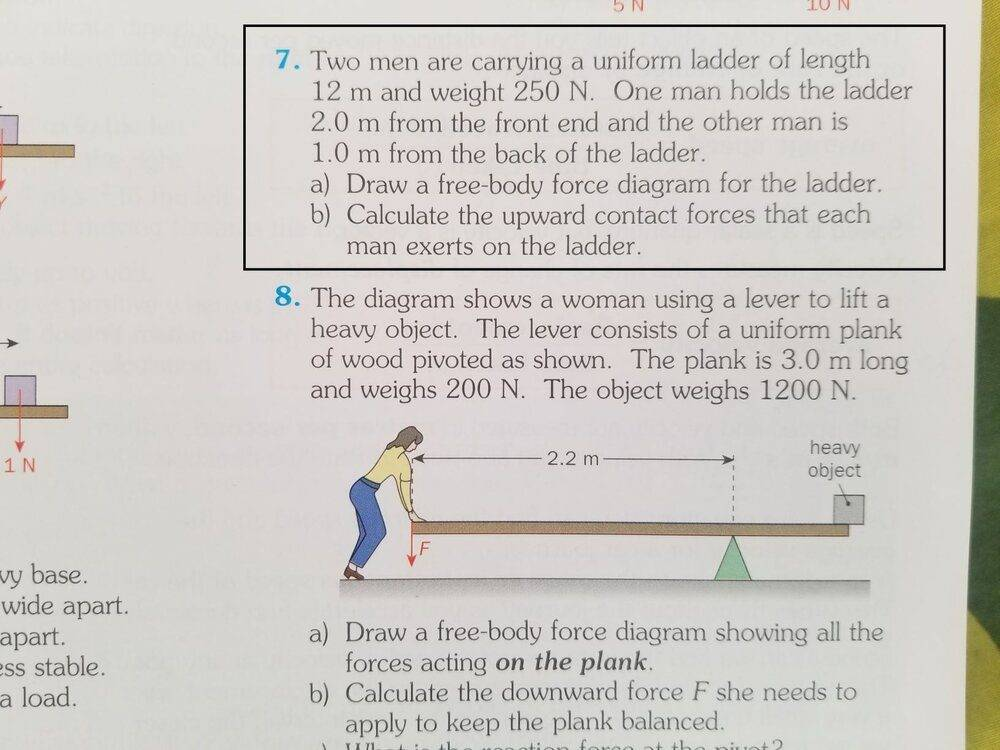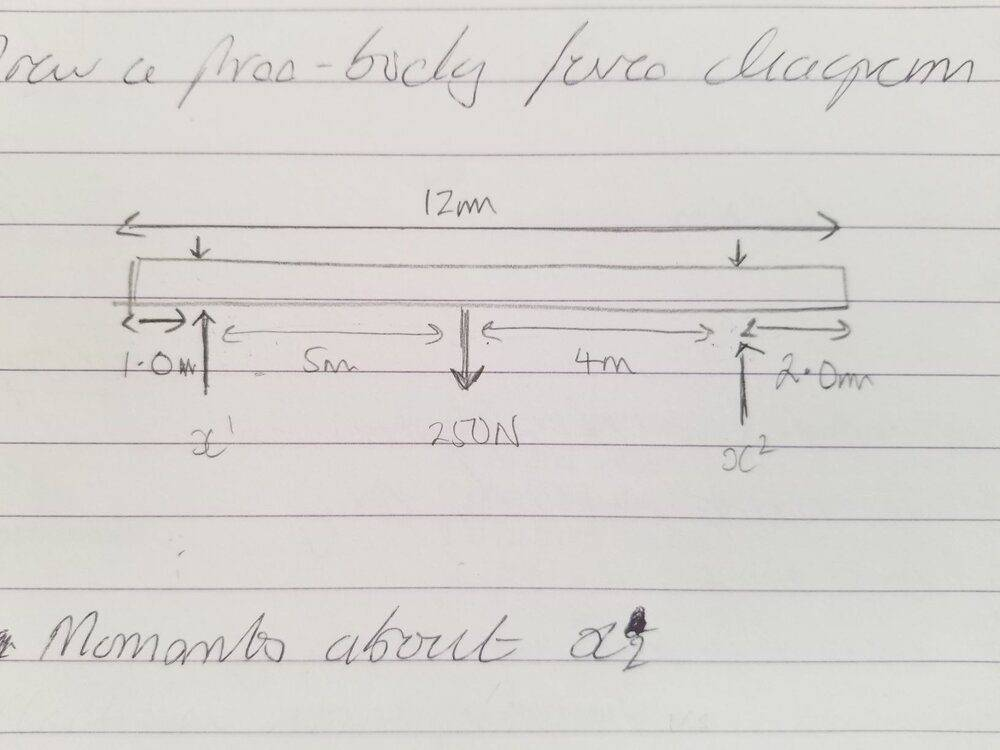# Calculating moments about a support point

Homework Statement:
I am required to calculate the upward contact forces that two men are exerting carrying a ladder horizontally at fixed points (question 7). I think I should calculating moments about each fixed point (where the men are carrying the ladder)
Relevant Equations:
Moment of Force = Force * Perpendicular distance
Moment about X2 to calculate force at X1:

x1 * 9 = (250 * 2)
Therefore, x1 = 500/9 = 55.5N

The book however gives force at x1 as 110N. So I figured I have not understood a concept somewhere•Delta2

Delta2
Homework Helper
Gold Member
Your mistake seems simple to me and it makes me wonder. Why do you take the torque (moment) of weight of the ladder as ##250N\cdot 2m##? This is wrong. What's the distance of the point of application of weight (Center of mass, CoM) from the point X2? Therefore what is the correct torque of weight?

•Shaye
Thanks Delta2. Sorry I got confused. I didn't think about the CoM/CoG where all the weight is acting. But now it's fine :-) The sum of the moments about the CoM should total 250N

Delta2
Homework Helper
Gold Member
Thanks Delta2. Sorry I got confused. I didn't think about the CoM/CoG where all the weight is acting. But now it's fine :-) The sum of the moments about the CoM should total 250N
Er the sum of moments about the CoM/CoG should total zero. Since we have equilibrium, the sum of moments about any point is zero.

Er the sum of moments about the CoM/CoG should total zero. Since we have equilibrium, the sum of moments about any point is zero.

The books solution says to take moments about x2 to find the force of x1 as 110N to 2 significant figures. Then by equilibrium: X1 + X2 = 250N therefore x2 = 250N - 110N = 140N.

I understood this to be (4/9)*250 = 110 2 s.f.

Similar to the reasoning of a couple i.e. (Couple = F*d) for example the forces acting on each end (in opposite directions) of a 1 meter ruler being 12N will produce a couple equal to 12N e.g.

Couple
12N*1 = 12Nm

Moments should always sum to the couple
(12*0.5)+(12*0.5) = 12 Nm
(12*0.4)+(12*0.6) = 12 Nm etc...

In answer to your question in post #2. That was a complete mistake. I was calculating the moment incorrectly assuming the ladder was on a pivot and in equilibrium and trying to find a balancing figure for the other force.

Delta2
Homework Helper
Gold Member
I understood this to be (4/9)*250 = 110 2 s.f.
That is correct. So the moment of weight about x2 is equal to 4x250.
Similar to the reasoning of a couple
I don't understand what you find similar to the moment of a couple, the forces we have here are not a couple.

•Lnewqban
That is correct. So the moment of weight about x2 is equal to 4x250.

I don't understand what you find similar to the moment of a couple, the forces we have here are not a couple.
I mean what I found similar was the fact that you could take moments about any point on the ruler example e.g. at [0.2,0.8], [0.4.0.6] etc. and when you add the moments up they should always equal the couple...

Lnewqban
Gold Member
I mean what I found similar was the fact that you could take moments about any point on the ruler example e.g. at [0.2,0.8], [0.4.0.6] etc. and when you add the moments up they should always equal the couple...
There is a dfference between the concepts of couple of forces and balance of moments.

https://en.m.wikipedia.org/wiki/Couple_(mechanics)

https://en.m.wikipedia.org/wiki/Torque#Static_equilibrium

You could visualize the situation shown by the problem like two men, holding a 9-meter long bar horizontally (there is balance of vertical forces), inducing no rotation onto it (there is balance of moments).

A concentrated weight of 250 Newtons is free to slide along the bar.
If the sliding weight is located exactly midway, each man is holding 125 N.
If the weight slides all the way to one end, one man is holding 250 N and the second man 0 N.

•Delta2